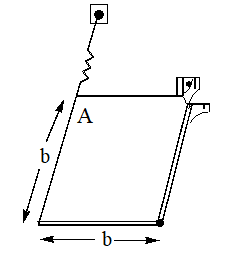A uniform square plate of mass m is supported in a horizontal plane by a vertical pin at B and is attached at A to a spring of constant K. If corner A is given a small displacement and released, determine the period of the resulting motion

# A uniform square plate of mass m is supported in a horizontal plane by a vertical pin at B and is attached at A to a spring of constant K. If corner A is given a small displacement and released, determine the period of the resulting motion1. A

$2\pi \sqrt{\frac{2m}{3k}}$

2. B

$2\pi \sqrt{\frac{m}{6k}}$

3. C

$\pi \sqrt{\frac{m}{3k}}$

4. D

$\sqrt{\frac{m}{3k}}$

Register to Get Free Mock Test and Study Material

+91

Verify OTP Code (required)

### Solution:

[Step 1]:  $\tau =I\alpha$
[Step 2]:  $Fb=\frac{2}{3}m{b}^{2}\alpha$
[Step 3]:  $-kbx=\frac{2m{b}^{2}\alpha }{3}$
[Step 4]:

[Step 5]:  $\omega =\sqrt{\frac{3k}{2m}}$
[Step 6]:  $T=2\pi \sqrt{\frac{2m}{3k}}$Register to Get Free Mock Test and Study Material

+91

Verify OTP Code (required)